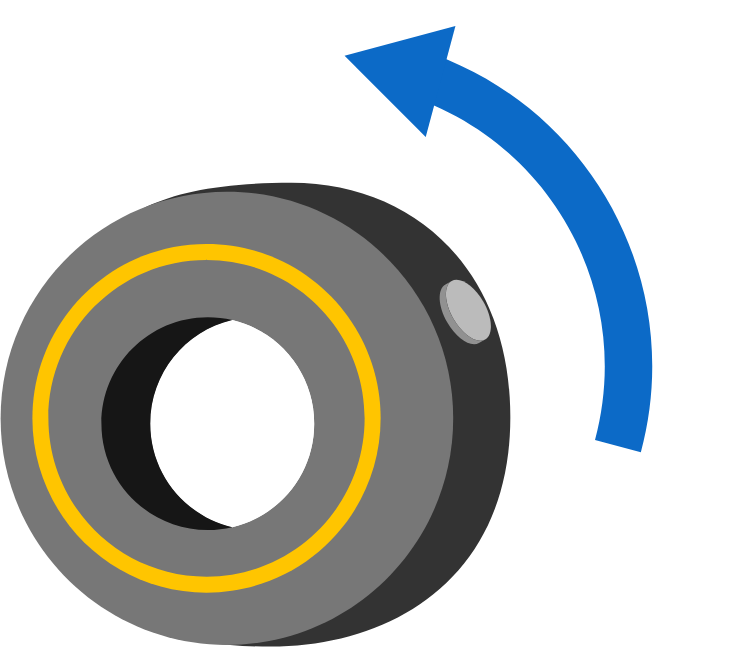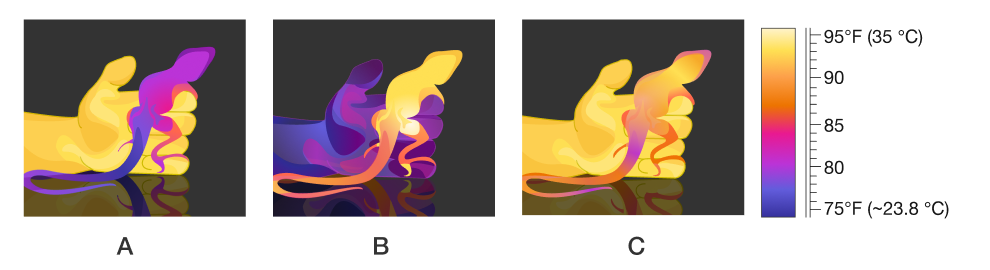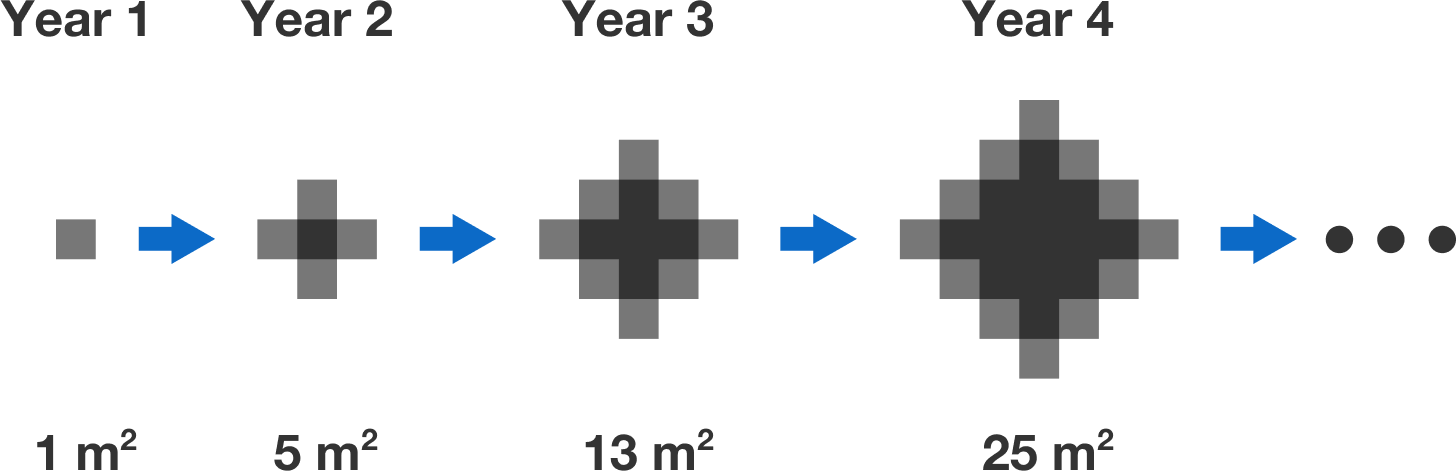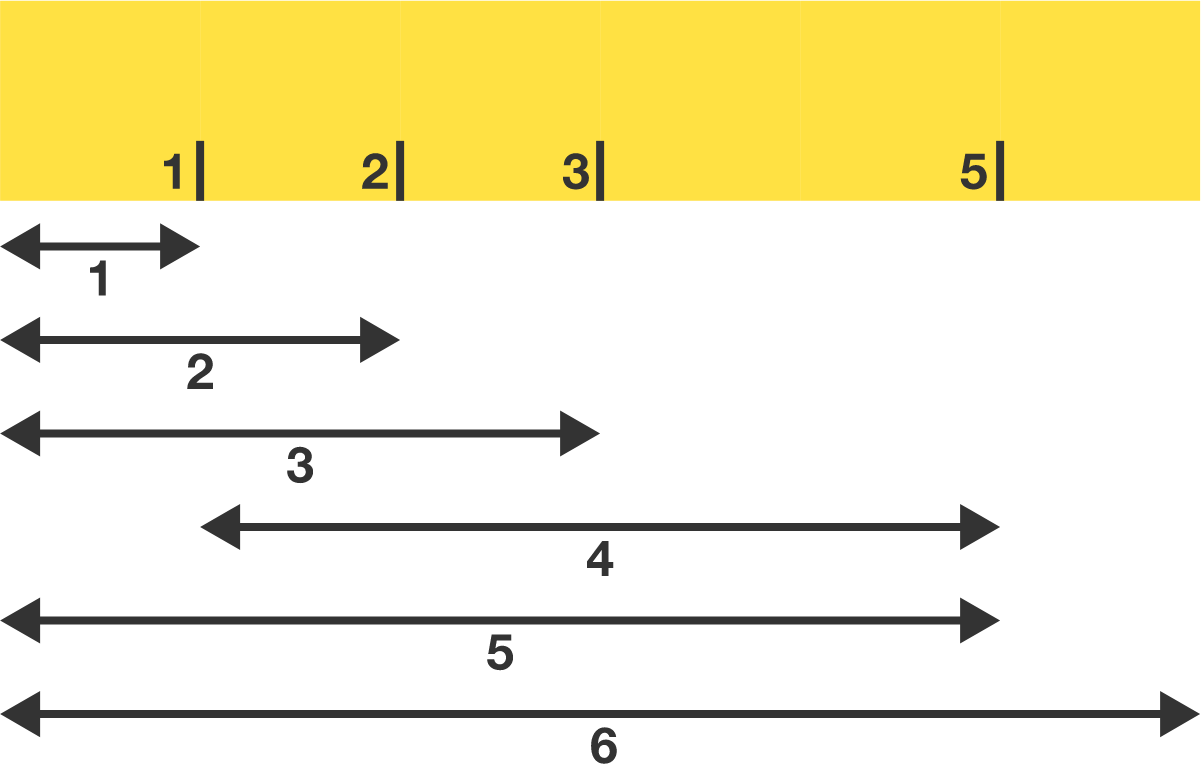# Problems of the Week

Contribute a problem

# 2018-03-19 Basic

A large nail is stuck in a tire, which is set in motion and left to roll on its own. Assuming there is sufficient friction for the tire to roll without slipping, what best describes the motion of the tire?A thermographic camera creates images based on infrared radiation. Which illustration most closely resembles a real thermographic photograph taken soon (within 10 seconds) after a gecko crawls onto a human hand on a night that is in the mid 70s (~24-25°C)?A sinkhole begins to open up in a deserted country. In the first year, the sinkhole is a $1\text{ m}$ square, and every year afterwards it grows an additional $1\text{ m}$ square from each of its outer edges.Steve notices the following pattern with the sinkhole area: \begin{aligned} 0^2+1^2 &= 1 \\ 1^2+2^2 &= 5 \\ 2^2+3^2 &= 13 \\ 3^2+4^2 &= 25. \end{aligned} Is the sinkhole area always the sum of the squares of two consecutive integers?

$a, b, c,$ and $d$ are positive integers such that $ab=cd$.

Can $a+b+c+d$ be a prime number?

Chris has an old 6-inch ruler that is missing the $4 \text{ in}$ mark. However, he can still measure all integer lengths from $1 \text{ in}$ to $6 \text{ in}.$ He'd like to see if he can erase more marks and still be able to measure all integer lengths from $1 \text{ in}$ to $6 \text{ in}.$

What is the minimum number of marks the ruler could have?Chris' ruler can measure all integer lengths from $1 \text{ in}$ to $6 \text{ in}$ with only 4 marks.

Note that all measurements must be made directly using the ends of the ruler and/or its marks. For example, you can't repeatedly use a distance of $1 \text{ in}$ to make larger distances.

×